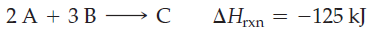×
Get Full Access to Introductory Chemistry - 5 Edition - Chapter 8 - Problem 72p
Get Full Access to Introductory Chemistry - 5 Edition - Chapter 8 - Problem 72p

×

# Consider the generic reaction: Determine the amount of heat emitted when each amount ofISBN: 9780321910295 34

## Solution for problem 72P Chapter 8

Introductory Chemistry | 5th Edition

• Textbook Solutions
• 2901 Step-by-step solutions solved by professors and subject experts
• Get 24/7 help from StudySoup virtual teaching assistantsIntroductory Chemistry | 5th Edition

4 5 1 271 Reviews
11
5
Problem 72P

Consider the generic reaction:Determine the amount of heat emitted when each amount of reactant completely reacts (assume that there is more than enough of the other reactant).

(a) 2 mol A

(b) 3 mol A

(c) 3 mol B

(d) 5 mol B

Step-by-Step Solution:

Solution 72P

Here, we are going to determine the amount of heat emitted when each amount of reactant completely reacts.

Step 1:

The given reaction is:

2A + 3B → C                 ΔHrxn = -125 kJ

The negative ΔHrxn indicates that the reaction is exothermic, i.e., heat is released during the reaction. Thus, the above reaction can be written as:

2A + 3B → C + 125 kJ(heat)                -----(1)

Step 2 of 2

##### ISBN: 9780321910295

Unlock Textbook Solution